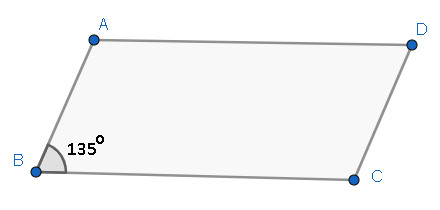Courses
Courses for Kids
Free study material
Offline Centres
MoreLast updated date: 24th Nov 2023
Total views: 279.3k
Views today: 8.79k

# In a parallelogram ABCD, if $\angle B=135{}^\circ$ , determine the measures of its other angles.Verified
279.3k+ views
Hint: We have to apply the properties of parallelogram to find the angles. We will use the property that the sum of adjacent angles in a parallelogram is supplementary, to find the measure of adjacent angles of B. Then, we will use the property that opposite angles in a parallelogram are equal, to find the measure of the remaining angles.

Complete step-by-step solution:
We are given with a parallelogram ABCD and $\angle B=135{}^\circ$ . We have to find the measures of its other angles.We know that in a parallelogram, the sum of adjacent angles supplementary. We write this mathematically as
$\Rightarrow \angle A+\angle B=180{}^\circ$
Let us substitute the given value of $\angle B$ in the above equation.
$\Rightarrow \angle A+135{}^\circ =180{}^\circ$
We have to take $135{}^\circ$ to the RHS.
\begin{align} & \Rightarrow \angle A=180{}^\circ -135{}^\circ \\ & \Rightarrow \angle A=45{}^\circ \\ \end{align}
We know that in a parallelogram, opposite angles are equal.
\begin{align} & \Rightarrow \angle B=\angle D=135{}^\circ \\ & \Rightarrow \angle A=\angle C=45{}^\circ \\ \end{align}
Hence, the measures of the angles are $\angle A=45{}^\circ ,\angle B=135{}^\circ ,\angle C=45{}^\circ ,\angle D=135{}^\circ$.

Note: Students must deeply learn the properties of parallelograms to solve these types of questions. We can also solve this question in an alternate method.
We have used the supplementary angle property in angles A and B. We can also apply this property to angles B and C.
$\Rightarrow \angle C+\angle B=180{}^\circ$
Let us substitute the given value of $\angle B$ in the above equation.
$\Rightarrow \angle C+135{}^\circ =180{}^\circ$
We have to take $135{}^\circ$ to the RHS. We will get
\begin{align} & \Rightarrow \angle C=180{}^\circ -135{}^\circ \\ & \Rightarrow \angle C=45{}^\circ \\ \end{align}
Now, we can use the opposite angle property to find the other angles similar to the above solution.